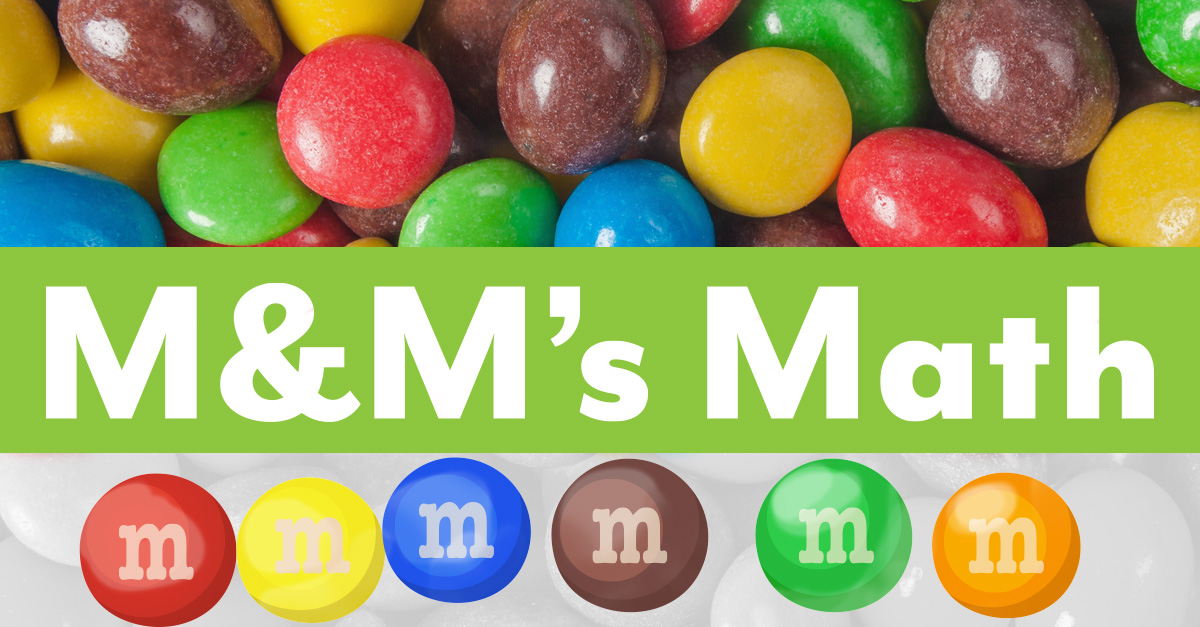# M&M’s MathThose tiny, candy-coated chocolates are more than just a tasty treat. M&M’s also are a great math manipulative to reinforce common math concepts like estimation, addition, multiplication, graphing, and so much more! These fun-filled activities take math skills to the next level while incorporating M&M’s into each lesson.

Estimation
Fill a jar with M&M’s and ask your children to estimate the number of M&M’s.

Sorting
Have your child sort a pile of M&M’s into color groups.

Counting
Tally the various color groups of M&M’s.

Sort a bowl of M&M’s into color groups. Then, use the candy to solve various operational problems to solve depending on age and skill level.

Blue + Yellow =
Yellow - Red =
Red x Green x Blue =
Yellow / Blue =
(Red + Green) x (Yellow – Blue) =
2(Red + Green) x (Yellow – Blue) x 5 =

Word Problems
Solve the word problems below or create your own.

1. Ruth has 6 more M&M’s than Mary. Mary has 9 M&M’s. How Many M&M’s does Ruth have?

2. Luke has 25 blue M&M’s and 42 green M&M’s. How many more green M&M’s are there than blue?

3. John has 126 green M&M’s and 89 brown M&M’s. Rachel took 42 of John’s green M&M’s. How many M&M’s does John have left?

Mean, Median, and Mode
A pile of M&M’s can be used to practice finding averages, middle numbers, and the number occurring most often.

Mean – The average of a data set.
Mode – The most common number in a data set.
Median – The middle of the set of numbers in sequential order.

1. Count the number of each individual color and record those results.

2. Count the total number of M&M’s and record the number.

3. Find the mean by adding up all the numbers. Then, divide by the numbers you added.

4. Rewrite the numbers from smallest to biggest and find the middle number. If there are two numbers in the middle, find the average of the two.

5. Find the mode by identifying the number that occurs the most.

6. Find the range by calculating the difference between the largest value and smallest value.

Fractions, Decimals, and Percentages
Use a pile of M&M’s to decide the fractional part of each color of M&M as compared to the entire group. For example, if you have 5 red M&M’s of a total group of 25, the fraction would be 5/25, which can be reduced to 1/5. Transfer the fractions to decimals and percentages as well.

Graphing
Sort a bag of M&M’s by color groups and record the numbers in a table. Use the information to create pictographs, bar graphs, comparison graphs, pie graphs, and more.

Measurement
Use the M&M’s as measuring tools to find the length, width, circumference, radius, perimeter, and/or area of various items around the house.